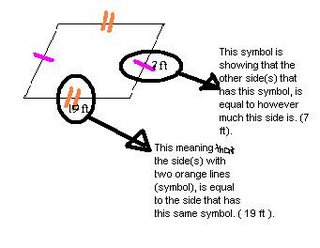## Wednesday, April 05, 2006

### April's Scribe Notes

Today in math class, we did another mad minute page in our booklets. It was basically questions about fractions, order of operations, and the B.E.D.M.A.S stuff. Mr. Reece gave about 2-3 minutes to finish.

Anyway, we also continued talking over about perimeter and asked questions such as "how can you find the perimeter of a
shape?". Well... all you need to do is add up all the sides of the shape to get the sum of the measurement.

We needed to know th
at information to help us finish yesterday's homework where we had to make 4 quares of our own that would equal one of the 4 measurements. One of them was 26cm. This is what it would look like:To do that you would have to get a shape and palce numbers 1 to 9 on each side to make it add up to the given measurements. The numbers hve to be on the opposite side of the shape too.
Height is the vertical side of the rectangle and width is horizontal.

Oh yeah, Mr. Reece handed out some dot sheets where we had to make as many rectangles as a group. The number of line segments were either 20, 24, or 30 and the maximum rectangles were 9,11, and 14. The width and height couldn't be as high or wide as 9 or 1.

That's pretty much it. Hopefully, I covered most of the today's class. But I'm going to let..............
MARK be the next scribe... just cause' he wants to! =)

## Monday, April 03, 2006

### Scribe Notes for April 3, 2006

Cjay's Scribe Notes:

In class today, we started off by Mr. Reece introducing us to the new mental math booklet. We had 3 minutes to complete Minute #2. Minute #2 included some of the previous work we did in the last term or two. (Order of Operations, multiplying, and so on.)
Then Mr. Reece introduced us to something called Perimeter. The perimeter of an object is the outside of the object. We found a rule for finding the perimeter of a object/polygon. The rule is to add up all the sides, (of the object), and that will give you the outside of the object, (the perimeter).
Mr. Reece drew an object/polygon on the smartboard, and we had to find out the perimeter of the object/polygon. All the sides of this object were equal, and were measured 18 mm. Another way to show this, was to label the measurement on one side of the object and make a symbol, and then copy the same symbol onto the other sides, (indicating that they are all equal). One of my fellow students helped Mr. Reece out by indicating that you don't always have to add up the sides, if they're all equal. You can just multiply the measurement by how many sides there are.Then Mr. Reece had an object on the smartboard, and the sides that were parallel / opposite of each other were equal. We were to find the perimeter, (with or without our group members' help).
Then Mr. Reece put a polygon on the overhead. This polygon was a triangle. The triangle had no equal sides, and it measured out to be 12 cm. One of this triangles' sides was measured 5 cm. The other; 4 cm, and last; 3 cm, which is a Scalene triangle.
Then on the smartboard, we were to find the missing measurement of a polygon. This polygon was a rectangle. The side at the top, was measured 30 mm. The left & right side, measured 8 mm. However, the bottom side, was unknown. Mr. Reece also gave us the total perimeter, which was 76 mm. We were to figure the missing measurement out. Eventually, everyone found out that the missing measurement was 30 mm. One way to figure that out is:
1) The top side was labeled 30 mm, and the top side is parallel / the opposite of the bottom side, which was unknown.
&
2)You can also add up the sides that are labeled, and subtract it from the total perimeter. That will give you the total perimeter.
And to end our day, we had a little assignment for homework. We were given 4 perimeter. We were to create a shape for each given perimeter. We had to draw a polygon, (any polygon, and not more than once), and it's sides must all equal the given perimeter. The sides don't have to be equal, just as long as the sum of the sides, is the perimeter.
This is a little like something we did in class:Except, we used 'cm' & 'mm', not 'Ft'.
That is mostly what we covered today in math class. If I missed any important points, I am SO sorry! =
Okee dokee, the next scribe will be... (eeny meeeny miiney Moe.) I pick April. (: Have fun April! :P